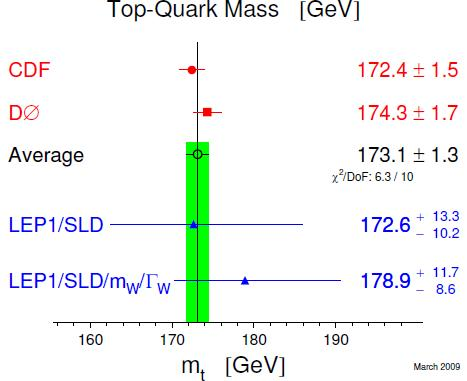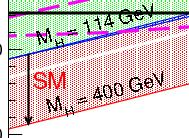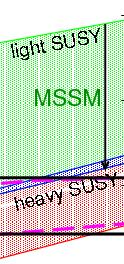## Some recent posts you might want to readMarch 6, 2010

Posted by dorigo in Blogroll, internet, news, physics, science.
Tags: , , , , , , , ,

As the less distracted among you know, I have moved my blogging activities to scientific blogging last April. I wish to report here a list of interesting posts I have produced there in the course of the last few months (precisely, since the start of 2010). They are given in reverse chronological order and with zero commentary – come see if you are curious.

## Latest global fits to SM observables: the situation in March 2009March 25, 2009

Posted by dorigo in news, physics, science.
Tags: , , , , , , , , , ,

A recent discussion in this blog between well-known theorists and phenomenologists, centered on the real meaning of the experimental measurements of top quark and W boson masses, Higgs boson cross-section limits, and other SM observables, convinces me that some clarification is needed.

The work has been done for us: there are groups that do exactly that, i.e. updating their global fits to express the internal consistency of all those measurements, and the implications for the search of the Higgs boson. So let me go through the most important graphs below, after mentioning that most of the material comes from the LEP electroweak working group web site.

First of all, what goes in the soup ? Many things, but most notably, the LEP I/SLD measurements at the Z pole, the top quark mass measurements by CDF and DZERO, and the W mass measurements by CDF, DZERO, and LEP II. Let us give a look at the mass measurements, which have recently been updated.

For the top mass, the situation is the one pictured in the graph shown below. As you can clearly see, the CDF and DZERO measurements have reached a combined precision of 0.75% on this quantity.The world average is now at$M_t = 173.1 \pm 1.3 GeV$. I am amazed to see that the first estimate of the top mass, made by a handful of events published by CDF in 1994 (a set which did not even provide a conclusive “observation-level” significance at the time) was so dead-on: the measurement back then was$M_t=174 \pm 15 GeV$! (for comparison, the DZERO measurement of 1995, in their “observation” paper, was$M_t=199 \pm 30 GeV$).

As far as global fits are concerned, there is one additional point to make for the top quark: knowing the top mass any better than this has become, by now, useless. You can see it by comparing the constraints on$M_t$ coming from the indirect measurements and W mass measurements (shown by the blue bars at the bottom of the graph above) with the direct measurements at the Tevatron (shown with the green band). The green band is already too narrow: the width of the blue error bars compared to the narrow green band tells us that the SM does not care much where exactly the top mass is, by now.

Then, let us look at the W mass determinations. Note, the graph below shows the situation BEFORE the latest DZERO result;, obtained with 1/fb of data, and which finds$M_W = 80401 \pm 44 MeV$; its inclusion would not change much of the discussion below, but it is important to stress it.Here the situation is different: a better measurement would still increase the precision of our comparisons with indirect information from electroweak measurements at the Z. This is apparent by observing that the blue bars have width still smaller than the world average of direct measurements (again in green). Narrow the green band, and you can still collect interesting information on its consistency with the blue points.

Finally, let us look at the global fit: the electroweak working group at LEP displays in the by now famous “blue band plot”, shown below for March 2009 conferences. It shows the constraints on the Higgs boson mass coming from all experimental inputs combined, assuming that the Standard Model holds.I will not discuss this graph in details, since I have done it repeatedly in the past. I will just mention that the yellow regions have been excluded by direct searches of the Higgs boson at LEP II (on the left, the wide yellow area) and the Tevatron ( the narrow strip on the right). From the plot you should just gather that a light Higgs mass is preferred (the central value being 90 GeV, with +36 and -27 GeV one-sigma error bars). Also, a 95% confidence-level exclusion of masses above 163 GeV is implied by the variation of the global fit$\chi^2$ with Higgs mass.

I have started to be a bit bored by this plot, because it does not do the best job for me. For one thing, the LEP II limit and the Tevatron limit on the Higgs mass are treated as if they were equivalent in their strength, something which could not be possibly farther from the truth. The truth is, the LEP II limit is a very strong one -the probability that the Higgs has a mass below 112 GeV, say, is one in a billion or so-, while the limit obtained recently by the Tevatron is just an “indication”, because the excluded region (160 to 170 GeV) is not excluded strongly: there still is a one-in-twenty chance or so that the real Higgs boson mass indeed lies there.

Another thing I do not particularly like in the graph is that it attempts to pack too much information: variations of$\alpha$, inclusion of low-Q^2 data, etcetera. A much better graph to look at is the one produced by the GFitter group instead. It is shown below.In this plot, the direct search results are introduced with their actual measured probability of exclusion as a function of Higgs mass, and not just in a digital manner, yes/no, as the yellow regions in the blue band plot. And in fact, you can see that the LEP II limit is a brick wall, while the Tevatron exclusion acts like a smooth increase in the global$\chi^2$ of the fit.

From the black curve in the graph you can get a lot of information. For instance, the most likely values, those that globally have a 1-sigma probability of being one day proven correct, are masses contained in the interval 114-132 GeV. At two-sigma, the Higgs mass is instead within the interval 114-152 GeV, and at three sigma, it extends into the Tevatron-excluded band a little, 114-163 GeV, with a second region allowed between 181 and 224 GeV.

In conclusion, I would like you to take away the following few points:

• Future indirect constraints on the Higgs boson mass will only come from increased precision measurements of the W boson mass, while the top quark has exhausted its discrimination power;
• Global SM fits show an overall very good consistency: there does not seem to be much tension between fits and experimental constraints;
• The Higgs boson is most likely in the 114-132 GeV range (1-sigma bounds from global fits).

## Zooming in on the HiggsMarch 24, 2009

Posted by dorigo in news, physics, science.
Tags: , , , , , , , , ,Yesterday Sven Heinemeyer kindly provided me with an updated version of a plot which best describes the experimental constraints on the Higgs boson mass, coming from electroweak observables measured at LEP and SLD, and from the most recent measurements of W boson and top quark masses. It is shown on the right (click to get the full-sized version).

The graph is a quite busy one, but I will try below to explain everything one bit at a time, hoping I keep things simple enough that a non-physicist can understand it.

The axes show suitable ranges of values of the top quark mass (varying on the horizontal axis) and of the W boson masses (on the vertical axis). The value of these quantities is functionally dependent (because of quantum effects connected to the propagation of the particles and their interaction with the Higgs field) on the Higgs boson mass.

The dependence, however, is really “soft”: if you were to double the Higgs mass by a factor of two from its true value, the effect on top and W masses would be only of the order of 1% or less. Because of that, only recently have the determinations of top quark and W boson masses started to provide meaningful inputs for a guess of the mass of the Higgs.

Top mass and W mass measurements are plotted in the graphs in the form of ellipses encompassing the most likely values: their size is such that the true masses should lie within their boundaries, 68% of the time. The red ellipse shows CDF results, and the blue one shows DZERO results.There is a third measurement of the W mass shown in the plot: it is displayed as a horizontal band limited by two black lines, and it comes from the LEP II measurements. The band also encompasses the 68% most likely W masses, as ellipses do.

In addition to W and top masses, other experimental results constrain the mass of top, W, and Higgs boson. The most stringent of these results are those coming from the LEP experiment at CERN, from detailed analysis of electroweak interactions studied in the production of Z bosons. A wide band crossing the graph from left to right, with a small tilt, encompasses the most likely region for top and W masses.So far we have described measurements. Then, there are two different physical models one should consider in order to link those measurements to the Higgs mass. The first one is the Standard Model: it dictates precisely the inter-dependence of all the parameters mentioned above. Because of the precise SM predictions, for any choice of the Higgs boson mass one can draw a curve in the top mass versus W mass plane. However, in the graph a full band is hatched instead. This correspond to allowing the Higgs boson mass to vary from a minimum of 114 GeV to 400 GeV. 114 GeV is the lower limit on the Higgs boson mass found by the LEP II experiments in their direct searches, using electron-positron collisions; while 400 GeV is just a reference value.

The boundaries of the red region show the functional dependence of Higgs mass on top and W masses: an increase of top mass, for fixed W mass, results in an increase of the Higgs mass, as is clear by starting from the 114 GeV upper boundary of the red region, since one then would move into the region, to higher Higgs masses. On the contrary, for a fixed top mass, an increase in W boson mass results in a decrease of the Higgs mass predicted by the Standard Model. Also note that the red region includes a narrow band which has been left white: it is the region corresponding to Higgs masses varying between 160 and 170 GeV, the masses that direct searches at the Tevatron have excluded at 95% confidence level.The second area, hatched in green, is not showing a single model predictions, but rather a range of values allowed by varying arbitrarily many of the parameters describing the supersymmetric extension of the SM called “MSSM”, its “minimal” extension. Even in the minimal extension there are about a hundred additional parameters introduced in the theory, and the values of a few of those modify the interconnection between top mass and W mass in a way that makes direct functional dependencies in the graph impossible to draw. Still, the hatched green region shows a “possible range of values” of the top quark and W boson masses. The arrow pointing down only describes what is expected for W and top masses if the mass of supersymmetric particles is increased from values barely above present exclusion limits to very high values.

So, to summarize, what to get from the plot ? I think the graph describes many things in one single package, and it is not easy to get the right message from it alone. Here is a short commentary, in bits.

• All experimental results are consistent with each other (but here, I should add, a result from NuTeV which finds indirectly the W mass from the measured ratio of neutral current and charged current neutrino interactions is not shown);
• Results point to a small patch of the plane, consistent with a light Higgs boson if the Standard Model holds
• The lower part of the MSSM allowed region is favored, pointing to heavy supersymmetric particles if that theory holds
• Among experimental determinations, the most constraining are those of the top mass; but once the top mass is known to within a few GeV, it is the W mass the one which tells us more about the unknown mass of the Higgs boson
• One point to note when comparing measurements from LEP II and the Tevatron experiments: when one draws a 2-D ellipse of 68% contour, this compares unfavourably to a band, which encompasses the same probability in a 1-D distribution. This is clear if one compares the actual measurements: CDF$80.413 \pm 48 MeV$ (with 200/pb of data), DZERO$80,401 \pm 44 MeV$ (with five times more statistics), LEP II$80.376 \pm 33 MeV$ (average of four experiments). The ellipses look like they are half as precise as the black band, while they are actually only 30-40% worse. If the above is obscure to you, a simple graphical explanation is provided here.
• When averaged, CDF and DZERO will actually beat the LEP II precision measurement -and they are sitting on 25 times more data (CDF) or 5 times more (DZERO).

## An exam in Subnuclear PhysicsJanuary 9, 2009

Posted by dorigo in physics.
Tags: , , , , , , , ,
• Draw the strong and electromagnetic coupling constants as a function of$Q^2$, explain their functional dependence using feynman graphs of the corrections to the photon and gluon propagators, write their formula, and compute the value of the constants at$Q^2=M_Z^2$, given the values at$Q^2=1 MeV^2$ (QED) and$Q^2=1 GeV^2$ (QCD).